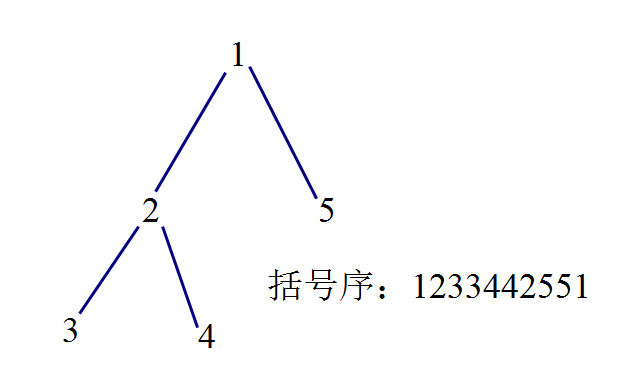# 莫队算法讲解（含树上莫队）

## 莫队算法

1、先对原序列进行分块。
2、离线操作，对询问进行排序，以左端点所在块编号第一关键字，右端点的位置第二关键字，进行排序。然后维护[l$l$,r$r$]的答案，并不断调整l$l$r$r$

1、左端点所在块编号确定时，右端点位置单调不下降，所以右端点移动最多造成的时间复杂度是O$O$(n$n$)的，总共n$\sqrt n$块，总时间复杂度为O$O$(nn$n\sqrt n$)。

2、左端点所在块编号进行变动时，右端点移动最多造成的时间复杂度是O$O$(n$n$)，总共n$\sqrt n$块，变动次数也就是n$\sqrt n$次，总时间复杂度为O$O$(nn$n\sqrt n$)。

3、块内左端点位置每次最多移动n$\sqrt n$，一共m$m$次询问，也就是一共移动m$m$次，总时间复杂度为O$O$(mn$m\sqrt n$)。

### 树上莫队（以下均遵循此原则，出现两次的数字不算入所求信息中）
1、如果j$j$k$k$的祖先，那么所求信息就为j$j$k$k$最后出现的位置之间的信息。
2、如果j$j$不是k$k$的祖先。那么所求信息就为j$j$最先出现的位置以及k$k$最后出现的位置之间的信息，我们发现，j$j$,k$k$lca$lca$不在其中，再把lca$lca$加上即可。

### 拓展：带修改的莫队©️2019 CSDN 皮肤主题: 精致技术 设计师: CSDN官方博客